# Texas Go Math Grade 3 Lesson 16.4 Answer Key Relate Figures, Fractions, and Area

Refer to our Texas Go Math Grade 3 Answer Key Pdf to score good marks in the exams. Test yourself by practicing the problems from Texas Go Math Grade 3 Lesson 16.4 Answer Key Relate Figures, Fractions, and Area.

## Texas Go Math Grade 3 Lesson 16.4 Answer Key Relate Figures, Fractions, and Area

Investigate

Materials; pattern blocks, color pencils, ruler

Connect Use what you know about combining and separating pattern blocks to explore the relationship between fractions and area.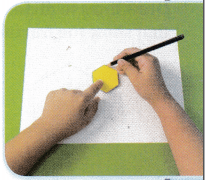A. Trace a hexagon pattern block.

B. Divide your hexagon into two parts with equal area. What new figures have you drawn?

C. Write the fraction that names each part of the whole you divided. __________
Each part is $$\frac{1}{2}$$ of the whole figure’s area.

D. Write the fraction that names the whole area. __________

Math Idea
Equal parts of a whole have equal area.The fraction that names the whole area.  $$\frac{1}{2}$$

Make Connections

The rectangle at the right is divided into four parts with equal area.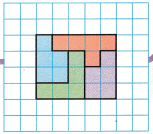• Write the unit fraction that names each part of the divided whole. ________
• What is the area of each part? ____________
• How many equal shares does it take to make one whole? ____________
• Does each equal share of the whole have the same shape? ____________
• Is the area of each equal share the same? Explain how you know.

• Write the unit fraction that names each part of the divided whole. $$\frac{1}{5}$$
• What is the area of each part? 5 square units
• How many equal shares does it take to make one whole? 4 equal shares
• Does each equal share of the whole have the same shape? No
• Is the area of each equal share the same? Explain how you know.
Yes, the area of each equal share is same

Explanation:
The rectangle is divided into 4 equal parts each part has equal area of 5 square units

Share and Show

Question 1.
Divide the trapezoid into 3 parts with equal area. Write the names of the new shapes. Then write the fraction that names the area of each part of the whole.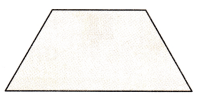Answer: trapezoid into 3 triangles with equal area.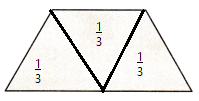Explanation:
The fraction that names the area of each part of the whole is $$\frac{1}{3}$$

Math Talk
Mathematical Processes

Explain how you know the areas of all the parts are equal.
If you add all the parts the total must be the whole
$$\frac{1}{3}$$ + $$\frac{1}{3}$$ + $$\frac{1}{3}$$ = $$\frac{3}{3}$$ = 1
Then that proves the areas of all the parts are equal

Draw lines to divide the figure into equal parts that show the fraction given.

Question 2.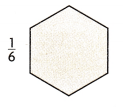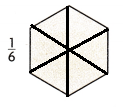Explanation:
Divided the figure into equal parts as the fraction given

Question 3.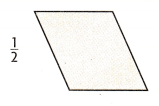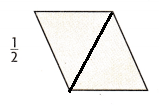Explanation:
Divided the figure into equal parts as the fraction given

Question 4.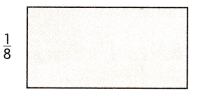Explanation:
Divided the figure into equal parts as the fraction given

Draw lines to divide the figure into parts with equal area. Write the area of each part as a unit fraction.

Question 5.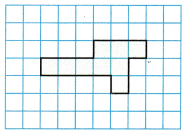3 equal parts ___________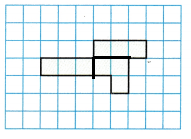Explanation:
Divided the figure into 3 equal parts as the unit fraction given

Question 6.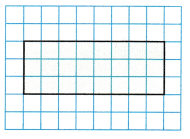6 equal parts ___________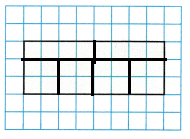Explanation:
Divided the figure into 6 equal parts as the  unit fraction given

Question 7.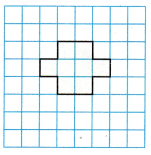4 equal parts ___________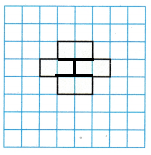Explanation:
Divided the figure into 4 equal parts as the unit fraction given

Problem Solving

Question 8.
H.O.T. Write Math If the area of three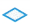is equal to the area of one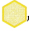, the area of how manyequals four? Explain your answer.
Explanation:The above figure shows how ais  divided into 3if the hexagons are four then 4 x 3 = 12

Question 9.
H.O.T. Apply Show how you can divide the hexagon into four shapes with equal area.Each part is _________ of the whole shape’s area.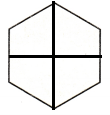Explanation:
Each part is $$\frac{1}{4}$$ of the whole shape’s area.

Question 10.
H.O.T. Multi-Step Sense or Nonsense?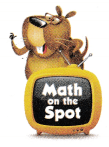Divide the hexagon into six equal parts.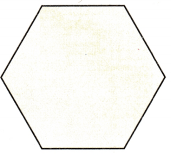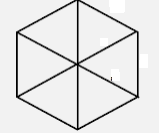Explanation:
Hexagon is divided into 6 equal parts
Which pattern block represents $$\frac{1}{6}$$ of the whole area?Explanation:
The shaded area represents $$\frac{1}{6}$$ of the whole area.

Divide the trapezoid into three equal parts.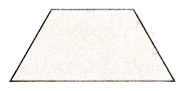Explanation:
Divided the hexagon into 3 equal parts
Which pattern block represents $$\frac{1}{3}$$ of the whole area?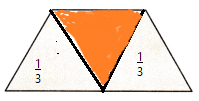Explanation:
The shaded part represents $$\frac{1}{3}$$ of the whole area.

Alexis said the area of $$\frac{1}{3}$$ of the trapezoid is greater than the area of $$\frac{1}{6}$$ of the hexagon because $$\frac{1}{3}$$ > $$\frac{1}{6}$$. Does her statement make sense? Explain your answer.
_______________________________________________

Write a statement that makes sense.Explanation:
Yes, her statement make sense
By the above figures we can say that her statement make sense

Question 11.
What if you divide the hexagon into 3 equal parts? Use Math Language to write a sentence that compares the area of each equal part of the hexagon to each equal part of the trapezoid.Explanation:
By the above figures a trapezoid is divided into 3 parts
and a hexagon to 3 parts fraction is same but area is different

Fill in the bubble for the correct answer choice.

Question 12.
Inez made a stained glass design with the rectangles shown at the right. Which rectangles have the same shape and the same area?(A) A and C
(B) B and D
(C) A and D
(D) B and C
Explanation:
A and D rectangles have the same shape and the same area.

Question 13.
Paulo buys a mirror. The frame is made of 6 same-size trapezoids. What unit fraction of the frame is each trapezoid?(A) $$\frac{1}{3}$$
(B) $$\frac{1}{6}$$
(C) $$\frac{1}{8}$$
(D) $$\frac{1}{2}$$
Answer: (B) $$\frac{1}{6}$$
Explanation:
$$\frac{1}{6}$$ unit fraction of the frame is each trapezoid.

Question 14.
Multi-Step Anastasia is painting one wall of her room. She divides the wall into B squares with the same area. She paints half of the squares light green and half of the squares dark green. What fraction of the wall is one dark green square?
(A) $$\frac{1}{2}$$
(B) $$\frac{1}{8}$$
(C) $$\frac{1}{4}$$
(D) $$\frac{1}{1}$$
Answer: (C) $$\frac{1}{4}$$
Explanation:
$$\frac{1}{4}$$ fraction of the wall is one dark green square

Texas Test Prep

Question 15.
Ray divided the rectangle at the right into six parts with equal area. What is the area of each part?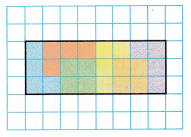(A) 6 square units
(B) 24 square units
(C) 4 square units
(D) 10 square units
Explanation:
area of each part is 4 square units

### Texas Go Math Grade 3 Lesson 16.4 Homework and Practice Answer Key

Draw lines to divide the figure into equal parts that show the fraction given.

Question 1.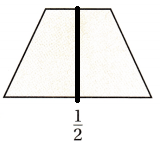Explanation:
Divided the figure into equal parts as the fraction given

Question 2.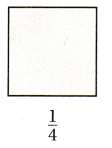Explanation:
Divided the figure into equal parts as the fraction given

Question 3.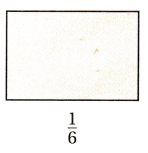Explanation:
Divided the figure into equal parts as the fraction given

Draw lines to divide the figure into parts with equal area. Write the area of each part as a unit fraction.

Question 4.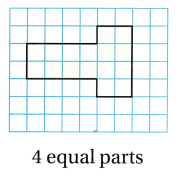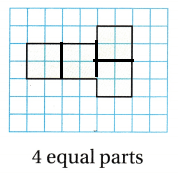Explanation:
Divided the figure into 4  equal parts as the unit fraction given

Question 5.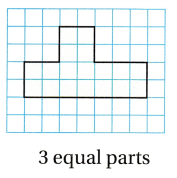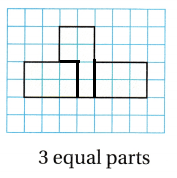Explanation:
Divided the figure into 3 equal parts as the unit fraction given

Problem Solving

Question 6.
Sanchez puts a window in his house made of 2 same-size triangles. What unit fraction of the window is each triangle?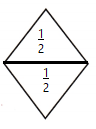Explanation:
Sanchez puts a window in his house made of 2 same-size triangles.
$$\frac{1}{2}$$ unit fraction of the window is each triangle

Lesson Check

Question 7.
Georgie has a rectangular window that is divided into 6 same-size squares. What fraction of the window is 1 square?
(A) $$\frac{1}{2}$$
(B) $$\frac{1}{4}$$
(C) $$\frac{1}{3}$$
(D) $$\frac{1}{6}$$
Answer: (D) $$\frac{1}{6}$$
Explanation:
$$\frac{1}{6}$$ fraction of the window is 1 square.

Question 8.
Marcy has a square window that is divided into 8 same-size squares. What fraction of the window is 1 square?
(A) $$\frac{1}{3}$$
(B) $$\frac{1}{4}$$
(C) $$\frac{1}{8}$$
(D) $$\frac{1}{2}$$
Answer: (C) $$\frac{1}{8}$$
Explanation:
$$\frac{1}{8}$$ fraction of the window is 1 square.

Question 9.
Rory divides the rectangle into 7 parts with equal area. What is the area of each part?(A) 4 square units
(B) 5 square units
(C) 7 square units
(D) 6 square unitsExplanation:
the area of each part is 6 square units

Question 10.
Multi-Step Perry makes a quilt with same-size squares. He makes 2 red squares, 2 blue squares, 2 yellow squares, and 2 green squares. What fraction of the quilt are the red squares?
(A) $$\frac{1}{4}$$
(B) $$\frac{1}{2}$$
(C) $$\frac{1}{8}$$
(D) $$\frac{1}{3}$$
Answer: (A) $$\frac{1}{4}$$
Explanation:
$$\frac{1}{4}$$ fraction of the quilt are the red squares

Question 11.
Multi-Step Doreen makes a painting with 12 same-size squares. She has painted one-half of the squares with a design. How many squares has Doreen painted?
(A) 1
(B) 4
(C) 3
(D) 6